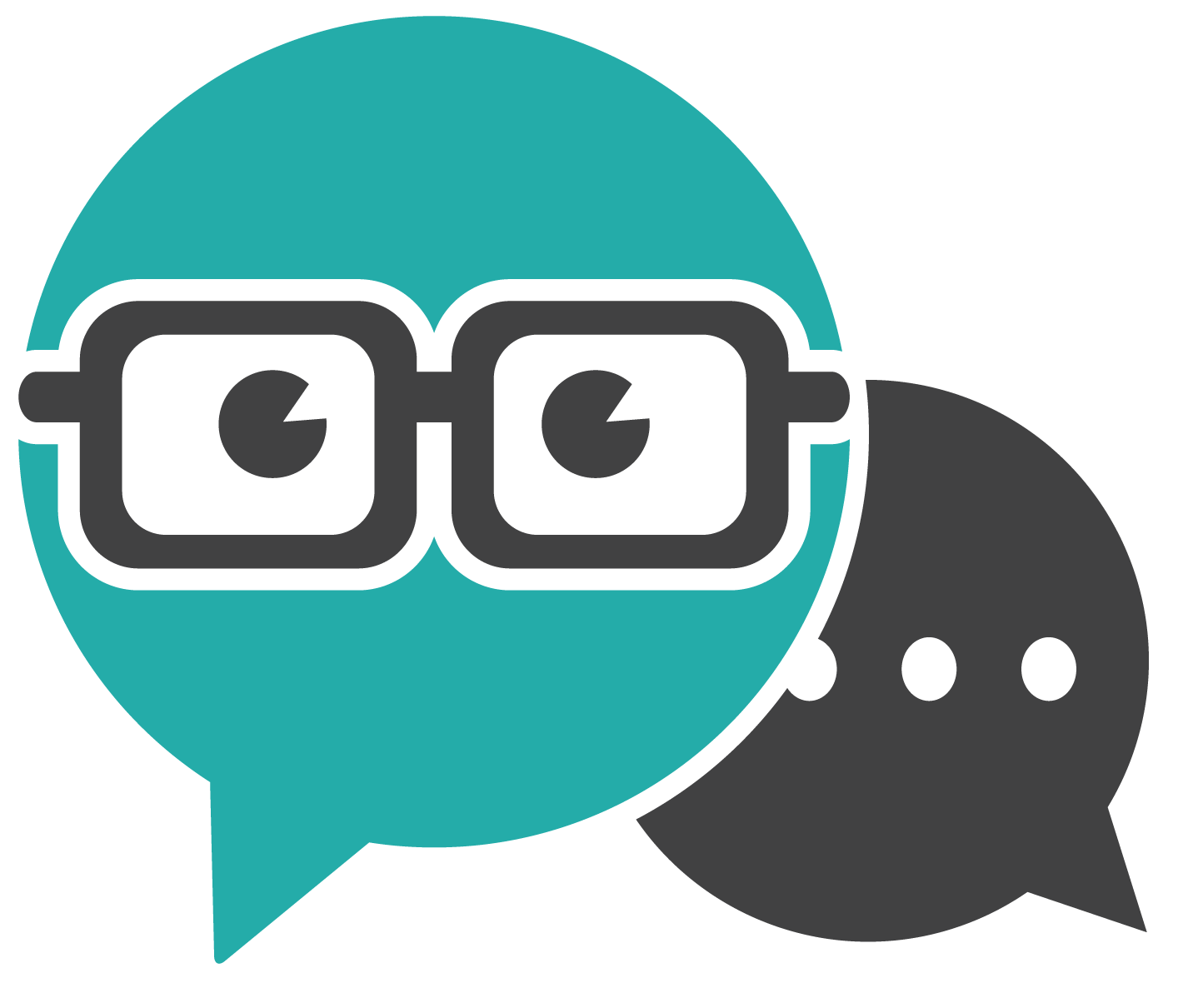Virtual Help

• Chat with library staff now

Math Language

Most of math is logic, but in order to work out the solution to a problem or understand an expression, you need to understand the words that are used. This module will explain the math terms that you will see in math, and will show you how to approach math word problems.

This module will help you understand, review and become comfortable with the language of math. To build math skills and confidence, it is important to be familiar with the basic words of math. Many terms are used throughout several areas of math, and it may be assumed you already know the meaning of these words. Some of these words look and sound similar, but have different meanings. Understanding the correct meaning of each term can help you to complete math problems.

This module contains:

• a glossary of commonly used math words and an example of each term
• a detailed description and examples of frequently used verbs in math exercises
• a strategy to approach and solve word problems
• activities to test your knowledge

Use this module to:

• Review math terms and test your knowledge
• Look up a word you don’t know the meaning of
• Understand what a math exercise is asking you to do
• Develop a strategy to apply to word problems
• Build confidence through practice exercises